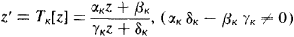# Automorphic Function

(redirected from Factor of automorphy)
Also found in: Wikipedia.
The following article is from The Great Soviet Encyclopedia (1979). It might be outdated or ideologically biased.

## Automorphic Function

in mathematics, an analytic function whose values do not change if its argument is subjected to certain linear fractional transformations. Automorphic functions include periodic functions and, in particular, elliptic functions.

For example, if the transformations are integer-valued and have the form of z’ = z + ω, where ω is a nonzero complex number, we obtain automorphic functions characterized by the equation f (z + ω) = f (z)—that is, periodic functions with period ω. In this case the transformation which does not modify the function is a shift of the plane by the vector ω. Obviously, the same shift repeated an arbitrary number of times will likewise not change the function. As a result we obtain a group of linear transformations z’ = z + n ω (n = 0, ±1, ±2, . . . ) which do not modify f (z). In the general case, assume that Γ is a group of linear fractional transformationsand that G is a region which is mapped onto itself by each of these transformations. Then a function f which is single-valued and analytic in G is an automorphic function, with respect to the group Γ, if f[TK (z)] = f (z) for k= 1, 2, . . . . The most important case is the one in which G is a circle or half-plane. Such a region may be regarded as the image of a Lobachevskii plane, and the transformations of Γ may be treated as motions in that plane. The corresponding automorphic functions may be regarded as such a generalization of periodic functions when shifts in a Euclidean plane are replaced by motions in a Lobachevskii plane. This point of view, developed by Poincaré, was the cornerstone of the successful construction of a general theory of automorphic functions. (Prior to Poincaré, important results in this field had been achieved by F. Klein.) In general, the entire theory of automorphic functions at present is a remarkable example of the fruitfulness of Lobachevskii’s geometrical concepts as applied to mathematical analysis and the theory of functions.

Besides problems of conformal mapping, other approaches to general automorphic functions are the theory of linear differential equations, the study of algebraic curves of order higher than the fourth, the solution of algebraic equations (for instance, the solution of general fifth-degree equations with one unknown involves automorphic functions), and others.

### REFERENCES

Ford, L. R. Avtomorfnye funktsii. Moscow-Leningrad, 1936. (Translated from English.)
Klein, F. Lektsii o razvitii matematiki v 19 stoletii, part 1. Moscow-Leningrad, 1937. Chapter 8. (Translated from German.)
Golubev, V. V. Lektsii po analiticheskoi teorii differentsial’nykh uravnenii, 2nd ed. Moscow-Leningrad, 1950.
Golubev, V. V. Odnoznachnye analiticheskie funktsii: Avtomorfnye funktsii. Moscow, 1961.